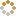﻿ Ningbo University of Technology Online Judge ::  Cirno's Trick
• ###  Cirno's Trick

• 时间限制: 1000 ms　内存限制: 65535 K
• 问题描述
•Cirno(チルノ) is an ice elf. Her hobby is tricking on others. But the biggest problem is that - Cirno is too foolish to do tricking. She knows this, so she makes statistics to calculate the probability that she can do tricking successfully.

Cirno writes down some probabilities that she may succeed. And she delete the lowest one, because it's too foolish and she can't believe in it. Then she delete the highest one, because she thinks it's impossible.
After that, she wants to know the average of the left probabilities.
• 输入
• This problem has several cases.
The first line of each case is an integer N (2 < N <= 1000), indicates the number of probabilities.
Then follows one line which containing N integers, indicate the probabilities. Each probability is a decimal between 0 and 100.
• 输出
• For each case, you should output the average of the left probabilities. Keep two decimal places.
• 样例输入
• ```3
0.0 50.0 100.0
```
• 样例输出
• ```50.00
```
• 提示
• ```Use 'double' to replace 'float'.
```
• 来源
• `XadillaX`
• 操作
﻿